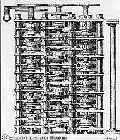p5.js FilesBabbage's Difference
Engine (circa 1832)

This folder contains some p5.js files that might be of interest. Included are some possibly useful programs, some demo files, and some how-to files related to p5.js.

The p5.js files have the file type .pjs. My editor has special features for different languages. It determines the language based on the file type which makes it desirable to distinguish between true Javascript .js files and p5.js files. In order to do so, the file type .pjs is being used for p5.js files.

The .pjs files assume the necessary p5.js include files are in "/p5js". If you decide to copy the files to your own computer, you may have to edit the names of those files in the <script> section.

These apts solve some common math and statistical problems both numerically and graphically

• A general purpose graphing program It allows rectangular, polar, and parametric functions. It includes some interesting examples. (Updated 3/17/2021 to allow an enlarged canvas option.)
• Solves f(x) = 0 using several different iterative methods including some that speed up iteration for multiple roots. It graphs the function and illustrates the iteration. Also can solve cubics analytically without iteration. (Significantly upgraded 11/12/2021.)
• diffiEq2 Solves 1st, 2nd order, and 3rd order initial value differential equations along with systems of up 3 differential equations numerically, plotting the results. It can also solve 2nd order boundary problems using a shooting method. If known, the analytical solution can also be plotted allowing one to compare the actual solution to the numerical solution. Four different numerical methods are provided. (Updated 5/6/2021 to allow an enlarged canvas option and some other options.)
• Charter Plots charts for line, column, pie, and scatter charts. It can also plot stacked column charts and combination column and line charts. Simple charts can be created easily but more sophisticated ones are possible. (Updated 8/10/2021.)
• Least Squares This program can do standard linear regression on a set of data points but can also fit non-linear curves for certain curves like y = exp(ax+b) and y = log(ax+b) that can be linearized.
• PolyRegression This apt does nonlinear regression analysis with 1st, 2nd, 3rd, and 4th degree polynomials. The results are graphed and some analysis is done to help determine which regression polynomial is "better". It is possible to enter data directly or from a file.
• Graphing converging Fourier Sine Series Helps illustrate the convergence of Fourier Series by graphing partial series sums for three common series.

Some more p5js code

• Linear system of equations Demo and test program for a function that solves linear equations.
• Solve nonlinear systems Solves systems of 1, 2, or 3 variables using Newton's method, an approximate Newton's method that doesn't require (partial) derivatives, or the secant method which also doesn't require derivatives.
• listMembers Three utility functions that list members of p5.js (and JavaScript) objects
• "How-To" Files relating to using p5.js
• CanvasClass CanvasClass allows one to easily use multiple canvases on the same web page without the difficulties of instance mode. Over 110 functions and event handlers are implemented.

The following two programs have been tested with Firefox, Chrome, Opera and the new Edge browsers. They may not work with the Edge Legacy browser. They have not been tested with Safari. The are not intended to be used and have not been tested on smart phones.

James Brink
Revised: 10/16/2021